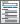Home  /  Products  /  Features  /  Survival analysis

## Survival analysis

Analyze duration outcomes—outcomes measuring the time to an event such as failure or death—using Stata's specialized tools for survival analysis. Account for the complications inherent in this type of data such as sometimes not observing the event (censoring), individuals entering the study at differing times (delayed entry), and individuals who are not continuously observed throughout the study (gaps). You can estimate and plot the probability of survival over time. Or model survival as a function of covariates using Cox, Weibull, lognormal, and other regression models. Predict hazard ratios, mean survival time, and survival probabilities. Do you have groups of individuals in your study? Adjust for within-group correlation using a random-effects or shared-frailty model.

Parametric survival modelsBayesian parametric survival modelsBayesian multilevel parametric survival modelsFinite mixtures of parametric survival models• Weibull, exponential, Gompertz, lognormal, loglogistic, or generalized gamma
• Both proportional-hazards and accelerated failure-time metrics
• Robust, cluster–robust, bootstrap, and jackknife standard errors
• Sampling weights and survey data
• PostestimationUtilities

Features of survival models• Single- or multiple-failure data
• Left-truncation
• Right-censoring
• Interval-censoring
• Time-varying regressors
• Gaps
• Recurring events
• Start–stop format
• Different types of failure events
• Customized time scales allowed
• Weibull, exponential, lognormal, loglogistic, or gamma model
• Robust, cluster–robust, bootstrap, and jackknife standard errors
• Weibull, exponential, lognormal, loglogistic, or gamma models
• Robust and cluster–robust standard errors
• Sampling weights and survey data
• Marginal predictions and marginal means
• Latent predictors of survival outcomes
• Path models, growth curve models, and more
• Weibull, exponential, lognormal, loglogistic, or gamma models
• Survival outcomes with other outcomes
• Sampling weights and survey data
• Marginal predictions and marginal means

Graphs of survivor, failure, hazard, or cumulative hazard functionUpdated

• View and run all postestimation features for your command
• Automatically updated as estimation commands are run

Obtain summary statistics, confidence intervals, etc.